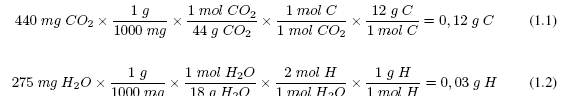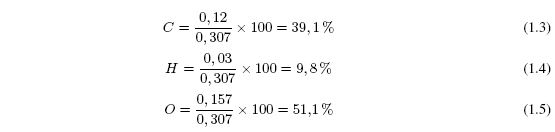It consists of experimentally determining the percentage composition (by mass) of a substance. Thus, for a compound with molecular formula C 4 H 10 the percentage composition is: 82.66% C and 17.34% H.
The elemental analysis of a compound is carried out in an apparatus, called an analyzer, where a weighed sample is burned with great precision (about 5 mg). Carbon is transformed into CO 2 , hydrogen into H 2 O and nitrogen into N 2 , determined by gas chromatography. Oxygen is not usually analyzed and is determined by difference.

Establish percent composition of a problem compound
For a determination on an old elemental analyzer, 307 mg of an organic compound are weighed. Its combustion originates 440 mg of CO 2 and 275 mg of H 2 O. Establish the percentage composition of the organic compound.Oxygen is calculated by difference 0.307-0.12-0.03 = 0.157 g O 2 . Now all that remains is to calculate the percentages of C, H and O.